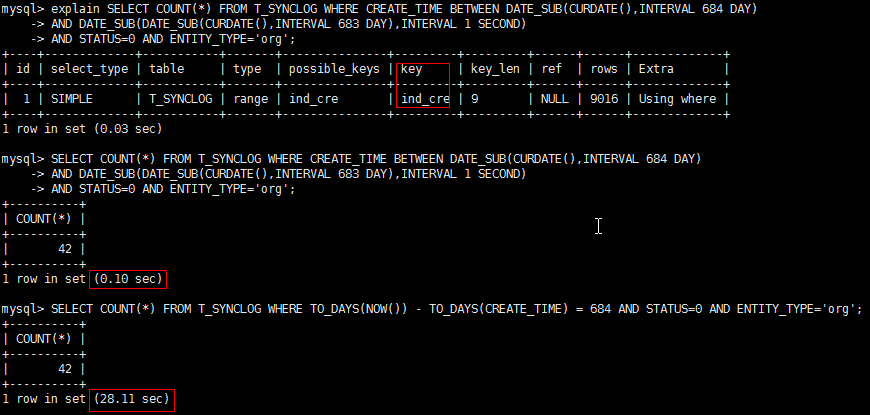# 针对where条件中日期型字段前面加函数的慢查询的优化

select count(*) from T_SYNCLOG where TO_DAYS(NOW()) - TO_DAYS(CREATE_TIME) = 0 and STATUS=0 and ENTITY_TYPE='org';

``````create index ind_cre on T_SYNCLOG (CREATE_TIME);

USE eip;
SELECT COUNT(*) FROM T_SYNCLOG WHERE CREATE_TIME BETWEEN CURDATE() AND NOW() AND STATUS=0 AND ENTITY_TYPE='org';

USE eip;
SELECT COUNT(*) FROM T_SYNCLOG WHERE CREATE_TIME BETWEEN DATE_SUB(CURDATE(),INTERVAL n DAY)
AND DATE_SUB(DATE_SUB(CURDATE(),INTERVAL n-1 DAY),INTERVAL 1 SECOND)
AND STATUS=0 AND ENTITY_TYPE='org';
``````THE END